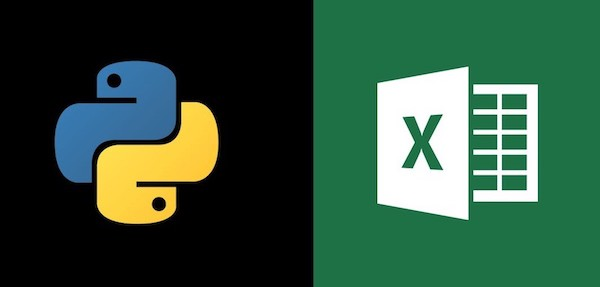## Automate python jobs by GitLab CI

Automating python scripts to run by GitLab CI (Runner) needs to configure with ".gitlab-ci.yml" with multiple parts like image, stage, only, manage environments and settings on GitLab.

Read more

## Python: Apply regular expression with regex

Regular expressions provide a flexible way to search or match string patterns in text. A single expression, commonly called a regex, is a string formed according to the regular expression language.Python’s built-in re module is responsible for applying regular expressions to strings. In this blog, I’ll first introduce regular expression syntax,...

Read more## How to manipulate excel workbook by Python?

This blog introduces how to use openpyxl package to manipulate Excel workbook and worksheet, like create a workbook and worksheet, write values in cells, set font(size, type, bold, italic), cut and paste values from one cell to another, remove one row, merge cells, set number format, set cells' background colors and center text in one cell, wrap...

Read more## Introduction to matplotlib.pyplot

In this blog, I will talk about some basic elements for creating a graph/graphs by package matplotlib.pyplot. Figures & Axes & Axis The figure can be seen as the canvas, on which all drawing components are plotted. The figure consists of axes, which are subdivisions of the figure. Each of the axes consists of one or more axis (horizonta...

Read more

## Introduction to pandas data structures

pandas provides high-level data structures and functions designed to make working with structured or tabular data fast, easy and expressive. In this blog I’ll introduce 2 workhorse data structures: Series and DataFrame. Series A Series is a one-dimensional array-like object containing a sequence of values and an associated array of data labels...

Read more

## Array manipulation - R vs Python

In this blog, I will talk about array manipulation via R and Python. You will see how to create an array, insert and delete an element from a 1d-array and a 2d-array. Creating arrays R dim1 <- c("one", "two", "three", "four") dim2 <- c("A", "B", "C") arr_2d <- array(1:12, dim = c(4, 3), dimnames = list(dim1, dim2)) > arr_2d ...

Read more

## Data structures - R vs Python

I’ve learnt python since the beginning of this year. In this blog, I’ll compare the data structures in R to Python briefly. Array R Atomic vectors one-dimensional array contain only one data type scalars are one-element vectors, e.g. f <- 3, g <- "US" function c() v <- c("k", "j", "w", "d", "v") > v  "k" > v[c(...

Read more